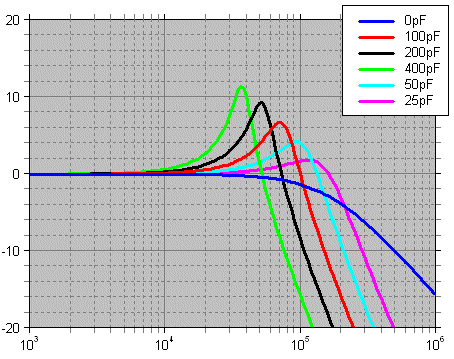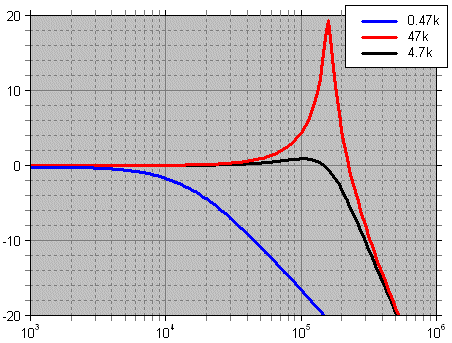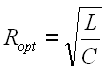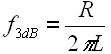This is a quick study of various phonograph cartridge loading conditions, a rather straightforward analysis using a simple model in Pspice. Obviously, real-life conditions are far more complex, but general trends and first order results can be realized. Effects of RIAA equalization have not been taken into account - perhaps altering the results a little.

The basic schematic is shown below. A cartridge's electrical response is simulated by a voltage source in series with an inductance (Lc) and resistance (Rc). The load capacitance of the interconnect and preamp are lumped together (Ca). Load resistance of preamp is Ra.Many moving magnet cartridges have inductances up to 500mH. Moving coils types range from 5uH to 5mH, (hence, the lower output voltage). The resistance of the windings is proportional to length (and number of turns). In the middle is the Grado "color" series of moving magnet cartridges which feature an inductance of about 50mH. For simulations I used examples of 500mH in series with 1000 ohms, 50mH & 100 ohms, and 5mH & 10 ohms. While this may not directly represent any particular cartridge, it offers some reasonable conditions to study.

The first thing we notice is that the circuit forms a basic LC resonant tank. Ignoring the effects of the resistances, the resonant frequency is given by:The larger the inductance, or larger the capacitance, the lower the resonant frequency will be. Indeed, it can easily show up in the audio band, even under common conditions. The following calculator can be used to determine the resonance of your system.

Inductance: mH, Capacitance: pF, Frequency = kHz

Looking at the "middle" cartridge (50mH & 100 ohms) into a 47k resistance, the loading capacitance was varied from 0pF to 400pF. Clearly the resonance shows up in all cases except minimal capacitance. The optimal load capacitance would seem to be about 10pF. That includes interconnect! Peaking can be reduced by lowering the 47k load resistance, but overall bandwidth will still be mainly determined by Lc and Ca.Ok, so where's the advantage of a moving coil? Here, the load was set at 47k and 200pF while the inductance was varied. The 500mH moving magnet type has a nice response but bandwidth is down 3dB at 20kHz. The moving coil (5mH) has terrific bandwidth, although the ultrasonic peak is quite pronounced.As mentioned earlier, the peaking is best damped by lowering the load resistance. This is why MC cartridge manufacturers often request loading of about 100 ohms. The next plot shows the 5mH 10 ohm cartridge loaded with 200pF and a variable resistance. Note the 4.7k loading provides very effective damping while maintaining a bandwidth of over 100kHz. Too much damping with the 470 ohm load pulls the response down too quickly. Most MC cartridges have less inductance than this example (chosen to highlight the issue), and so the typical loading value of 100 ohms is usually quite reasonable.So there you have it. Every system will be a little different and can be optimized for maximal bandwidth without peaking (flat response).

Ok, given the above analysis, it is pretty easy to calculate the proper load resistance for optimum tuning. It is simply:Using the previous L & C values:

Ropt = k

MC Cartridges

The super-low self-inductance of a moving coil cartridge puts them in their own category. At such levels, the loading capacitance becomes relatively insignificant, with system bandwidth now dominated by inductance and load resistance. This is now approximated by:Determine the bandwidth of your system using the following calculator:

Inductance: mH, Load Resistance: k, Bandwidth = kHz

Step-Up Transformers

This is a confusing subject for many because of the nonlinearity of the impedance transformation. Simply put, the impedance differs by the square of the turns ratio. For a 1:10 (20dB) step-up, the imedance is transformed by a factor of 100. That is, with the secondary loaded by 47k ohms, the primary reflects what appears to be 470 ohms to the cartridge.

Standard step-up ratios are 1:5 (14dB), 1:10 (20dB), 1:20 (26dB), and 1:30 (30dB). To find out the loading your cartridge sees, use the following calculator.